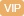| APP | 校园号 | 客服 客服热线：400-863-9889

400-863-9889（2017秋•扬州期末）分母是5的所有最简真分数的和是
2
2
，2里面有
32
32
 1 16
【考点】分数的加法和减法分数除法【专题】运算顺序及法则．
【分析】运用乘分配律进行计算．

【解答】解：7.×5.2+76×.8+36
=
 3 4
+
 1 4
÷
 1 6

=
 49 5
+
 499 5
+
 499 5
+
 1 5
+
 1 5
+
 1 5

=0.251
059×
 1 4
+02×25%0.2×0.25
=0.5
=2.25
=7.6×（5.+4.8）+6
113×
 1 4

 3 4
+025÷（
 2 3
-1.×
 1 3

=
 3 4
+
 1 4
÷（
 2 3
-
 3 2
×
 1 3

=（
 49 5
+
 1 5
）+（
 499 5
+
 1 5
）+（
 499 5
+
 1 5

=（85+6+）×
 1 4

=796
 49 5
+
 499 5
+
 499 5
+
 1 5
×3
=10+0+1000
85×
 1 4
26×
 1 4
+2×
 1 4

=2
 1 4
【点评】考查运算定简便运算，则混运算．注意运顺序运算法则，灵运用所学的运算简便计算

0/0